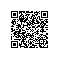# 我也说说Emacs吧(6) - Lisp速成

Lisp的含义是表处理语言。它的代码组成结构都是用括号组成的表来表示的。Lisp中的功能，要么是以函数形式求值，要么本身就是一些特殊表。

## Lisp基本函数速成

(message (concat "Hello" "," "World" "!"))

### 算术函数

#### 加法 +

(message "%d" (+ 1 1))

#### 减法 -

(message "%d" (- 1 1))

#### 乘法 *

(message "%d" (* 123456789 987654321))

(message "%d" (* 123456789 3145926535897932384626433832795928841971))

  debug(error (overflow-error "3145926535897932384626433832795928841971"))

#### 除法 /

(message "%d" (/ 128.1 3.0))

#### 求余 %

(message "%d" (% 128.1 3.0))

Debugger entered--Lisp error: (wrong-type-argument integer-or-marker-p 128.1)
%(128.1 3.0)
(message "%d" (% 128.1 3.0))
eval((message "%d" (% 128.1 3.0)))

#### 加1 1+

(message "%d" (1+ 1))

(message "%d" (+ 1 1))

#### 减1 1-

(message "%d" (1- 1))

### 数学函数

#### 求e的阶乘，exp函数

(message "%d" (exp 10))

#### 求阶乘函数 expt

(message "%d" (expt 2 8))

#### 求对数函数 log

(message "%d" (log 256 2))
(message "%d" (log 100))

#### 平方根函数 sqrt

(message "The square root of 128 is:%d" (sqrt 128))

"The square root of 128 is:11"

#### 求绝对值函数

(message "The absolute vaule of -1 is:%d" (abs -1))

The absolute vaule of -1 is:1

#### 三角函数

sin, cos, tan都是例行公事的函数，反函数是asin, acos, atan,

(message "%d" (sin 0))
(message "%d" (cos 0))
(message "%d" (tan 1))
(message "%d" (asin 1))
(message "%d" (acos 1))

### 逻辑运算

• 两数逻辑与 logand
• 两数逻辑或 logior
• 两数逻辑异或 logxor
• 两数逻辑非 lognot

(message "%d" (logand 1 0))
(message "%d" (logior 1 0))
(message "%d" (logxor 1 0))
(message "%d" (lognot 0))

### 逻辑运算特殊表

• 与 and: 对每个参数进行求值，直到遇上一个nil
• 或 or: 对每个参数进行求值，直到遇上一个非nil
• 非 not：not是nil别名。其实取反就是判断一个逻辑值是否为nil.

### 比较函数

• =: 等于
• >: 大于
• <: 小于
• >=: 大于或等于
• <=: 小于或等于
• /=: 不等于
• eq: 等于

(/= (logand 1 0) (lognot 1))

### 判断函数

• atom: 判断是不是一个原子
• listp: 判断是不是一个列表
• null: 判断是不是空
• stringp: 判断是不是一个字符串
• characterp: 判断是不是一个字符
• symbolp: 判断是不是一个符号
• zerop: 判断是不是0.
• intergerp: 判断是不是整数
(atom ())
(listp ())
(null ())
(stringp "Hello")
(characterp "h")
(symbolp nil)
(numberp 1)
(zerop 0)
(integerp 1)

## if特殊表

if特殊表的结构是(if 条件判断 THEN表 ELSE表 ... )

(if (atom ()) (message "() is an atom") (message "() is not an atom"))

### cond特殊表

cond特殊表的会一直执行后面的表，直至遇到任何一个表的值为非nil为止。

 (cond ((= x y) "x and y are the same")
((> x y) "x is greater than y")
((< x y) "x is less than y"))

## 定义函数 - defun特殊表

Emacs Lisp的函数定义要比Common Lisp多几样东西。因为我们前面讲了，emacs lisp的函数就是我们正常调用的命令，所以它要定义交互方式，要定义函数文档。不过好在这两部分都是可选的，完全不知道的情况下，仍然能写出可以正确执行的函数来。

emacs lisp的defun特殊表的格式如下:
(defun 函数名 (形参列表) "可选的函数文档" (interactive;可选的交再继续方式)

(defun evenp (x)
(if (= 0 (% x 2)) t
nil))
(evenp 2)

(defun evenp (x)
"Check if x is an even number or not"
(if (and (integerp x) (= 0 (% x 2))) t
nil))

(defun set-mark-command (arg)
...
(cond ((eq transient-mark-mode 'lambda)
(kill-local-variable 'transient-mark-mode))
((eq (car-safe transient-mark-mode) 'only)
(deactivate-mark)))
(cond
((and (consp arg) (> (prefix-numeric-value arg) 4))
(push-mark-command nil))
((not (eq this-command 'set-mark-command))
(if arg
(pop-to-mark-command)
(push-mark-command t)))
((and set-mark-command-repeat-pop
(eq last-command 'pop-global-mark)
(not arg))
(setq this-command 'pop-global-mark)
(pop-global-mark))
((or (and set-mark-command-repeat-pop
(eq last-command 'pop-to-mark-command))
arg)
(setq this-command 'pop-to-mark-command)
(pop-to-mark-command))
((eq last-command 'set-mark-command)
(if (region-active-p)
(progn
(deactivate-mark)
(message "Mark deactivated"))
(activate-mark)
(message "Mark activated")))
(t
(push-mark-command nil))))

## 小结

• if特殊表：用来进行判断
• cond特殊表：多分支判断
• and特殊表：求值直到遇见nil为止
• or特殊表：求值直到遇见非nil为止
• defun特殊表：用来定义函数使用钉钉扫一扫加入圈子
+ 订阅